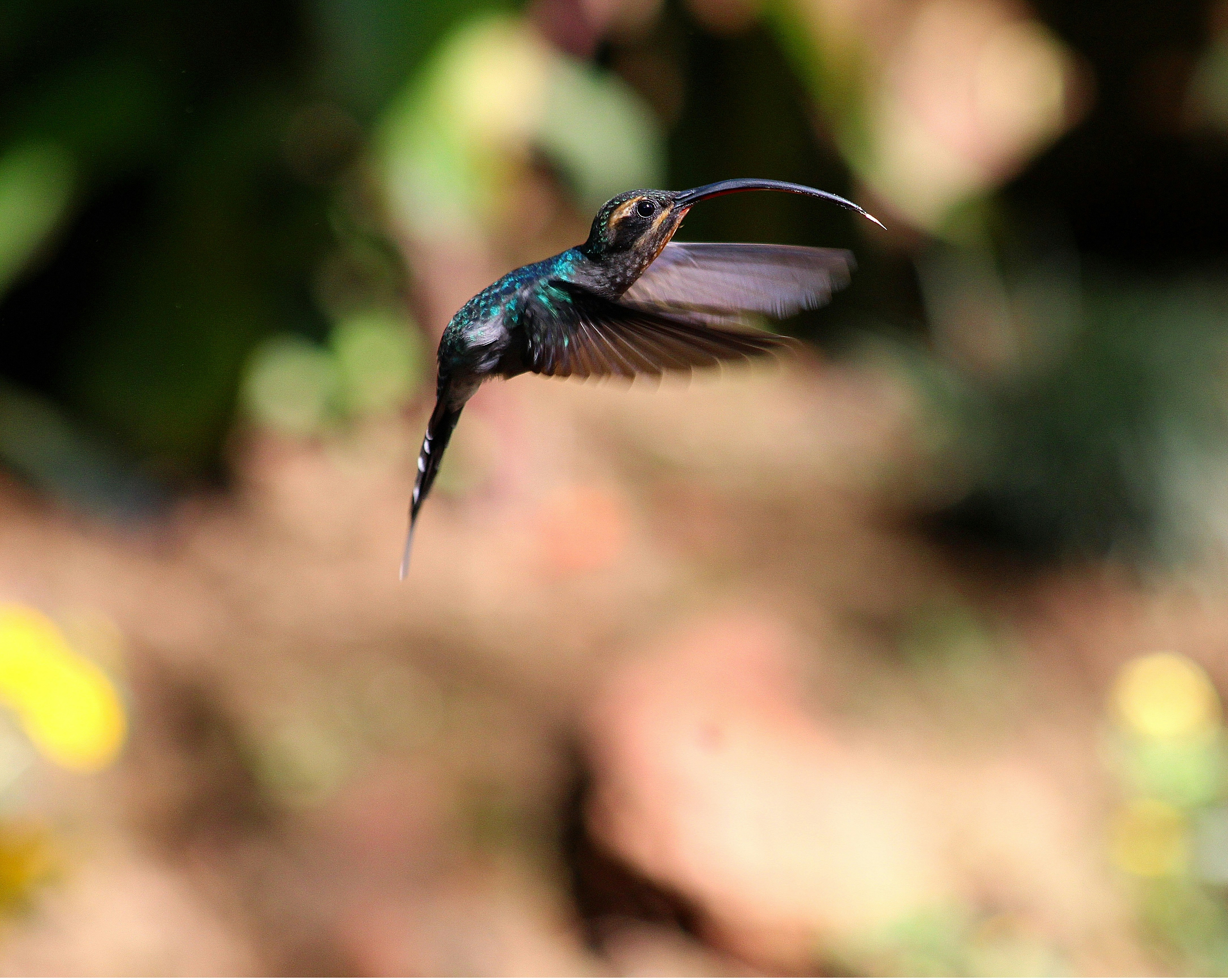0

# Dart for Java Devs #1Let's jump right in!

Go to https://dartpad.dartlang.org/ for online compilation.

We'll build a simple Dart class. Similar to Java: Bicycle class

Above the

``main()``
``Bicycle``
class with three instance variables. Also remove the contents from
``main()``
, as shown in the following code snippet:

``````class Bicycle {
int speed;
int gear;
}

void main() {
}``````
• Dart's main method is named
``main()``
.
• In Dart, you can define code outside of classes. Variables, functions, getters, and setters can all live outside of classes.
• The original Java example declares private instance variables using the private tag, which Dart doesn't use.
• "Neither main() nor Bicycle is declared as public, because all identifiers are public by default. Dart doesn't have keywords for public, private, or protected.
• Dart uses two-character indentation by convention instead of four.

## Add the following constructor to the Bicycle class:

``Bicycle(this.cadence, this.speed, this.gear);``
• This constructor has no body, which is valid in Dart.
• If you forget the semicolon (
``;``
) at the end of a no-body constructor, Dart displays the following error: "A function body must be provided.
• "Using
``this``
in a constructor's parameter list is a handy shortcut for assigning values to instance variables.

The code above is equivalent to the following:

``````Bicycle(int cadence, int speed, int gear) {
this.speed = speed;
this.gear = gear;
}``````

## Instantiate and print a Bicycle instance

``````void main() {
var bike = Bicycle(2, 0, 1);
print(bike);
}``````
• If you know that a variable's value won't change, then you can use final instead of var.

Run it. The following output comes:

``Instance of 'Bicycle'``

## Improve the output

While the output "Instance of ‘Bicycle'" is correct, it's not very informative. All Dart classes have a

``toString()``
method that you can override to provide more useful output.

``````@override
String toString() => 'Bicycle: \$speed mph';
``````
• The
``@override``
annotation tells the analyzer that you are intentionally overriding a member. The analyzer raises an error if you failed to properly perform the override.
• Dart supports single or double quotes when specifying strings.
• Use string interpolation to put the value of an expression inside a string literal:
``\${expression}``
. If the expression is an identifier, you can skip the braces:
``\$variableName``
.
• Shorten one-line functions or methods using fat arrow (
``=>``
) notation.

You should see the following output:

``Bicycle: 0 mph``

The original Java example defines

``speed``
as a read-only variable—it declares it as private and provides only a getter. Next, you'll provide the same functionality in Dart.

To mark a Dart identifier as private to its library, start its name with an underscore (

``_``
). You can convert
``speed``

In the Bicycle constructor, remove the speed parameter:

``Bicycle(this.cadence, this.gear);``

In

``main()``
, remove the second (
``speed``
) parameter from the call to the
``Bicycle``
constructor:

``var bike = Bicycle(2, 1);``

Change the remaining occurrences of

``speed``
to
``_speed``
. (Two places)

Initialize

``_speed``
to 0:

``int _speed = 0;``

Add the following getter to the

``Bicycle``
class:

``int get speed => _speed;``

Observations

• Uninitialized variables (even numbers) have the value
``null``
.
• The Dart compiler enforces library privacy for any identifier prefixed with an underscore. Library privacy generally means that the identifier is visible only inside the file (not just the class) that the identifier is defined in.
• By default, Dart provides implicit getters and setters for all public instance variables. You don't need to define your own getters or setters unless you want to enforce read-only or write-only variables, compute or verify a value, or update a value elsewhere.
• The original Java sample provided getters and setters for
``cadence``
and
``gear``
. The Dart sample doesn't need explicit getters and setters for those, so it just uses instance variables.
`` bike.cadence``
, and later refactor it to use getters and setters. The API stays the same. In other words, going from a field to a getter and setter is not a breaking change in Dart.

Finish implementing speed as a read-only instance variable

Add the following methods to the Bicycle class:

``````void applyBrake(int decrement) {
_speed -= decrement;
}

void speedUp(int increment) {
_speed += increment;
}``````

The final code:

``````class Bicycle {
int _speed = 0;
int get speed => _speed;
int gear;

void applyBrake(int decrement) {
_speed -= decrement;
}

void speedUp(int increment) {
_speed += increment;
}

@override
String toString() => 'Bicycle: \$_speed mph';
}

void main() {
var bike = Bicycle(2, 1);
print(bike);
}``````

The final Dart example looks similar to the original Java, but is more compact at 23 lines instead of 40: